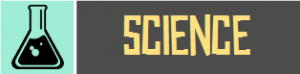# Grade 12 – Review and Exam Week#### Grade 12 English Teacher Hanan Ali

Vocabulary
– 20,000 Leagues Under the Sea Vocabulary Words
– Farmer and the Viper Vocabulary Words

Grammar
– Suffixes and Prefixes
– Subordinating Conjunctions to Express Cause and Effect

Recognize Sequence
– Plot Structure
– Sequence of Events

Comprehension
– Narrator Point of View
– Recognizing Motifs#### Grade 12 Physical Science II Teacher Ian Innocenti

1. Introduction to waves
2. Different types of waves
3. Properties of waves
4. EM waves
5. Behavior of light waves
6. Sound waves
7. Properties of sound waves

7.1 Chemical Names and Formulas
Using the periodic table of elements to locate the names and formulas of elements and to identify its corresponding ions, default charges, and its classification whether as a monatomic or diatomic element
Using the periodic table of elements to determine the atomic number, mass number, group number and the number of electrons, protons and neutrons

7.2 Oxidation Numbers
Using skills in basic algebra to compute the assigned oxidation numbers of elements in a compound

7.3 Using Chemical Formulas
Calculation of molar mass of a compound with the aid of periodic table
The use of appropriate units in calculating molar masses
Calculation of percentage composition using basic math skills

7.4 Determining Chemical Formulas
Understanding the difference between molecular formula and empirical formula
Solving empirical formula problems

8.1 Describing Chemical Reactions
Understanding the signs in a chemical reaction
Predicting the nature of reactants and products in a simple reaction

8.2 Types of Chemical Reactions
Understanding the five types of chemical reactions and balancing of equations
Predicting the reactants and products of chemical reactions

8.3 Activity Series
Understanding the nature of activity series and its problems
Predicting the occurrence of a reaction

9.1 Introduction to Stoichiomentry
Understanding stoichiometry and mole ratios
Solving mole ratio and mass composition problems

9.2 Ideal Stoichiometric Calculations
Understanding the molar mass of reactants and products
Solving molar mass from the given reactants and products

9.3 Limiting Reactant and Percentage Yield
Understanding the nature of a limiting reactant and knowing the percentage yield
Solving limiting reactant and percentage yield problems

10 The Kinetic-Molecular Theory of Matter
Introduction to kinetic-molecular theory of matter
Applications of Kinetic-Molecular Theory of Matter to Solids, and LiquidsSection A: Multiple Choice (40 Marks)
Mixture of questions on work covered during the term

Section B: Calculations (30 Marks)

Section C: Short Questions (30 Marks)
Mix of short answer questions (1-5 points) about the work covered during the term

#### Grade 12 Calculus Teacher Nic Hansen

Applications of Differentiation
– Extrema on an Interval
– Rolle’s Theorem
– Mean Value Theorem
– Increasing and Decreasing functions
– First Derivative Test
– Concavity
– Inflection Points
– Second Derivative Test

Previous Weekly Summaries